#### BramLabs

Joined Nov 21, 2013
98
Hello againI want to ask, if i made a capacitor bank with each cell of capacitor = 2.7V and 10 F.
And i made a circuit of capacitor bank like this :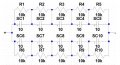So the total of voltage given by the circuit = 13.5V (2.7V + 2.7V + 2.7V + 2.7V + 2.7V)
And the total of capacity of the capacitor would be = Ctot = 4F ( 1/Ctot = (1/10 + 1/10 + 1/10 + 1/10 + 1/10) + (1/10 + 1/10 + 1/10 + 1/10 + 1/10 ) )
So, i have 13.5V and 4F capacitor bank.

What i wanna ask is that, if i put the capacitor bank in circuit like this :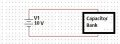The question is, what's the value of the LOAD RESISTANCE (in this case, the load resistance is the capacitor bank) ?

And if i measure with my multimeter like this :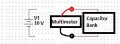and let's say i get 114 ohm. What kind of resistance is that ? The ESR (equivalent series of resistance) of the capacitor ? or the resistance of the capacitor bank (in this case is the thevenin of the 10k resistance) ? or what ?

Thank you before

#### Alec_t

Joined Sep 17, 2013
13,337
The load resistance is dynamic: it depends on the state of charge of the caps.If you connect your meter as shown, on the Ohms range, you could destroy it.

•BramLabs

#### ErnieM

Joined Apr 24, 2011
8,353
The load will carry from a dead short (when all caps are discharged) to 25K (when all caps are fully charged). Reading a steady 114 ohms indicates a problem somewhere.

The ESR will raise the dead short value slightly but not enough to really matter.

The caps will charge very rapidly, limited only by the output resistance of the battery, slightly more if you play the ESR into that.

•BramLabs

#### BramLabs

Joined Nov 21, 2013
98
The load resistance is dynamic: it depends on the state of charge of the caps.If you connect your meter as shown, on the Ohms range, you could destroy it.
The load will carry from a dead short (when all caps are discharged) to 25K (when all caps are fully charged). Reading a steady 114 ohms indicates a problem somewhere.

The ESR will raise the dead short value slightly but not enough to really matter.

The caps will charge very rapidly, limited only by the output resistance of the battery, slightly more if you play the ESR into that.
Oh sorry, i forgot to tell, i measure the capacitor bank when the capacitor is discharged. And i think it's not fully discharged because it wasn't 0 ohm, i left the supercapacitor for about 3 weeks, and i measured it and get 114 ohms.

Hmm... so basically, you guys are saying that the load resistance based on the state of capacitor (charged or discharged). So, if let's say we want to design some kind of circuit, with the a capacitor bank as a load, how to determine the load value ?
Let's just say, i want to design a buck converter with the capacitor bank as the load. In the equation, i need the value of R as the load.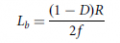So, what's the 'R' value based on my question with the capacitor bank as the load ?
If the value of the 'R' varied then, the value of inductor also would be varied T__T.

#### crutschow

Joined Mar 14, 2008
31,582
The value of "R" is not a parameter to use in your application.
If you tell us what you are trying to do instead of giving us bits and pieces, we could help you better.

•BramLabs

#### BramLabs

Joined Nov 21, 2013
98
The value of "R" is not a parameter to use in your application.
If you tell us what you are trying to do instead of giving us bits and pieces, we could help you better.
Okay sir, what i want to make is this one :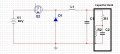And let's say the capacitor bank i've made is 13.5V and 4F. And for the safety, i want to charge the capacitor with 8V and current up to 3 Amps.

And as you can see the load would be R1, R2 and C2 as represent of the capacitor bank. R1 would be the ESR of the capacitor. R2 would be the passive balancing for the capacitor.
So, i think the load is the capacitor bank itself. And my question is, when designing the buck converter. There will be load parameter. And in this case, the load is capacitor bank. But the value of the load makes me confused, because there're capacitor in there and also the resistance (whether it's ESR or the passive balancing, i don't know), and both of them (capacitor and the resistance < ESR & passive balancing > ) are the load. So, which one is the load. And if the load are all of them, then how to design the buck converter with 'that' parameter.

Thanks before

Last edited:

#### Sensacell

Joined Jun 19, 2012
3,142
Be aware that supercaps in series may not have identical capacitance and will charge at unequal rates.
This can lead to over voltage conditions, which will damage the capacitor.

The 10 K resistors don't really help much here, you need an active clamp / shunt regulator to protect the caps.

•BramLabs

#### crutschow

Joined Mar 14, 2008
31,582
Okay, it's good we have a more complete picture of what you are trying to do.

The only resistance of interest is R1, the ESR and that is likely to be very low.

So if you want to charge the capacitor bank with good efficiency while limiting the current to 3A then you will need to design the buck regulator with current limiting in the switching control circuitry (which many IC control chips already have).
That way it can output no more than 3A into a short or low impedance (since that's essentially what the capacitor bank will look like until it is completely charged).

•BramLabs

#### BramLabs

Joined Nov 21, 2013
98
Be aware that supercaps in series may not have identical capacitance and will charge at unequal rates.
This can lead to over voltage conditions, which will damage the capacitor.

The 10 K resistors don't really help much here, you need an active clamp / shunt regulator to protect the caps.
Thank you sir for your concern. Yeah, that's true the capacitance itself aren't identical each other. In the design itself, i'll add a feedback mechanism voltage to the microcontroller to read the ADC of voltage (just using voltage divider) and if the value of the voltage more than the limit that i want, the pwm of the switch will turn off..
And yeah, 10k resistor doesn't really help so much, that's why it's called passive balancing. ahahhaha... But i just want to design something simple and easy, ahahaha... For another project, maybe i'll try an active balancing sir.
Thank you so much.

Okay, it's good we have a more complete picture of what you are trying to do.

The only resistance of interest is R1, the ESR and that is likely to be very low.

So if you want to charge the capacitor bank with good efficiency while limiting the current to 3A then you will need to design the buck regulator with current limiting in the switching control circuitry (which many IC control chips already have).
That way it can output no more than 3A into a short or low impedance (since that's essentially what the capacitor bank will look like until it is completely charged).
Wahahaha... actually i'm not making this circuit sir, i'm making a bidirectional converter. But in buck-mode, the circuit will look like the usual buck converter. So i think i need to understand the basic first. ahahaha...
Oh yeah, i wanna ask sir, is that a problem if i intend to charge the capacitor with more than 3A ? I mean, i know that the more current, then the hotter it will be, and because of it, i should aware of the others component. But let's just focus on the load, if the load only the capacitor with the ESR itself (without the passive balancing), is it okay to charge the capacitor more than 3A ? And how about the time of charging the capacitor, is it based on the voltage or based on the current ? (I think, based on the formula itself, it's based on the voltage + value of capacitance and the resistance, because Vc = V(1-e^(t/rc)) ).

Thank you sir ^^

#### crutschow

Joined Mar 14, 2008
31,582
How fast you can charge the capacitors is basically only limited by the capacitor surge current rating.

•BramLabs